Electron. J. Differential Equations, Vol. 2019 (2019), No. 18, pp. 1-16.

### Multiple solutions for discontinuous elliptic problems involving the fractional Laplacian Jung-Hyun Bae, Yun-Ho Kim

Abstract:
In this article, we establish the existence of three weak solutions for elliptic equations associated to the fractional Laplacian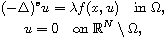where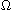is an open bounded subset in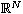with Lipschitz boundary,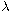is a real parameter, 0<s<1, N>2s, and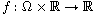is measurable with respect to each variable separately. The main purpose of this paper is concretely to provide an estimate of the positive interval of the parametersfor which the problem above with discontinuous nonlinearities admits at least three nontrivial weak solutions by applying two recent three-critical-points theorems.

Submitted April 26, 2018. Published January 30, 2019.
Math Subject Classifications: 58E30, 49J52, 58E05.
Key Words: Fractional Laplacian; three-critical-points theorem; multiple solutions.

Show me the PDF file (284 KB), TEX file for this article.

 Jung-Hyun Bae Department of Mathematics Sungkyunkwan University Suwon 16419, Korea email: hoi1000sa@skku.edu Yun-Ho Kim Department of Mathematics Education Sangmyung University Seoul 03016, Korea email: kyh1213@smu.ac.kr